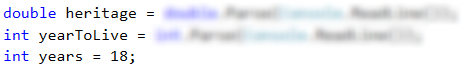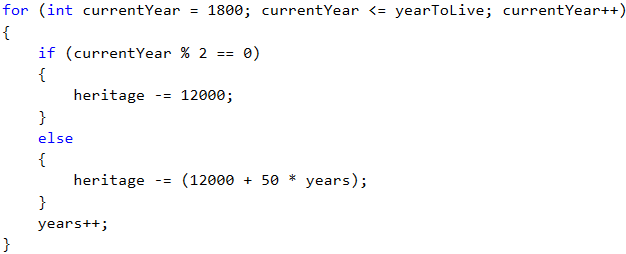# Problem: Back to the Past

Ivan is 18 years old and receives an inheritance that consists of X money and a time machine. He decides to return to 1800, but does not know if the money will be enough to live without working. Write a program that calculates if Ivan will have enough money to not have to work until a particular year (inclusive). Assuming that for every even (1800, 1802, etc.) year he will spend 12 000 dollars. For every odd one (1801, 1803, etc.) he will spend 12 000 + 50 * [the age he will have reached in the given year].

## Input Data

The input is read from the console and contains exactly 2 lines:

• Inherited money – a real number in the range [1.00 … 1 000 000.00].
• Year, until which he has to live in the past (inclusive) – integer number in the range [1801 … 1900].

## Output Data

Print on the console 1 line. The sum must be formatted up to the two symbols after the decimal point:

• If money is enough:
• "Yes! He will live a carefree life and will have {N} dollars left." – where N is the money that will remain.
• If money is NOT enough:
• "He will need {М} dollars to survive." – where M is the sum that is NOT enough.

## Sample Input and Output

Input Output Explanations
50000
1802
Yes! He will live a carefree life and
will have 13050.00 dollars left.

1800 → even
→ Spends 12000 dollars
→ Remain 50000 – 12000 = 38000
1801 → odd
→ Spends 12000 + 19*50 = 12950 dollars
→ Remaining 38000 – 12950 = 25050
1802 → even
→ Spends 12000 dollars
→ Remaining 25050 – 12000 = 13050

100000.15
1808
He will need 12399.85 dollars
to survive.

1800 → even
→ Remaining 100000.15 – 12000 = 88000.15
1801 → odd
→ Remaining 88000.15 – 12950 = 75050.15

1808 → odd → -399.85 - 12000 = -12399.85
12399.85 is not enough

## Hints and Guidelines

Let’s solve the problem step by step: read the input data, iterate over the years, check the heritage and print the output.

### Reading the Input Data

The method to solve this task is no different than the previous ones, so we start declaring and initializing the necessary variables:The requirements say that Ivan is 18 years old, so when declaring the variable years we assign it an initial value of 18. We read the other variables from the console.

### Iterating through the Years

Using a for loop, we will iterate through all years. We start from 1800 – the year in that Ivan returns, and we reach the year until which he must live in the past. We check in the loop if the current year is even or odd. We do this by division with remainder (%) by 2. If the year is even, we subtract from heritage 12000, and if is odd, we subtract from heritage 12000 + 50 * (years).### Checking for Enough Heritage and Printing the Output

Finally, we need to print out the results by checking if the heritage is enough to live without working or not. If the heritage is a positive number, we print: “Yes! He will live a carefree life and will have {N} dollars left.”, and if it is a negative number: “He will need {М} dollars to survive.”. Do not forget to format the sum up to the second digit after the decimal point.

Hint: Consider using the Math.Abs(…) function when printing the output, if the heritage is not enough.

## Testing in the Judge System

Test your solution here: https://judge.softuni.org/Contests/Practice/Index/511#2.